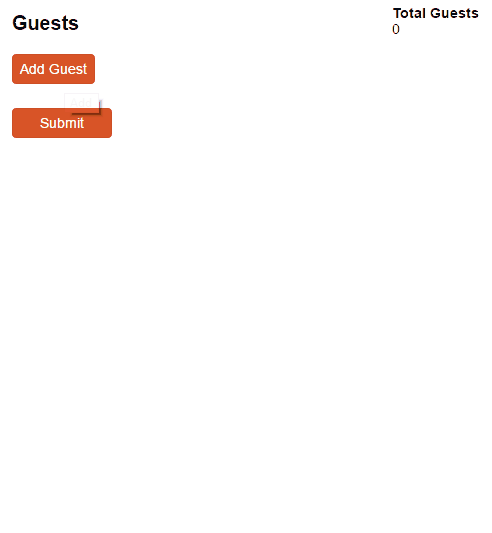# How do I total a repeating section?

To total the number of items in a repeating section, you can use the Count() function.

For example, let’s say you have a Party RSVP form that collects guest information in a repeating section. To calculate the total number of guests:

1. Add a Calculation field to your form – make sure it’s outside of your repeating section.
2. Use the Count() function to total the number of items: `=Guests.Count()`.
3. Now, the field will calculate the total number of guests.You can also add up specific fields inside repeating sections using the Sum function, with the field name in between the parentheses: `=Guests.Sum(PricePerGuest)`